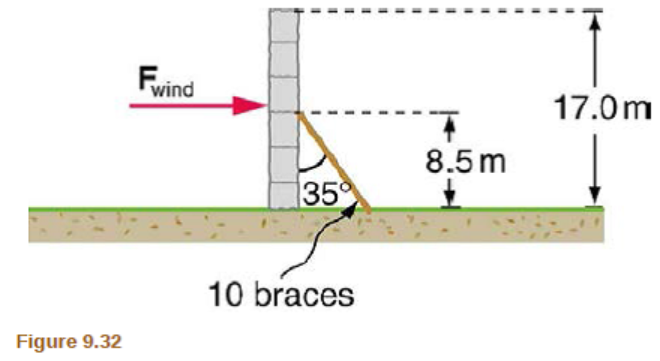# Problem: A 17.0-m high and 11.0-m-long wall under construction and its bracing are shown in Figure 9.32. The wall is in stable equilibrium without the bracing but can pivot at its base. Calculate the force exerted by each of the 10 braces if a strong wind exerts a horizontal force of 650 N on each square meter of the wall. Assume that the net force from the wind acts at a height halfway up the wall and that all braces exert equal forces parallel to their lengths. Neglect the thickness of the wall.

###### FREE Expert Solution

Torque:

$\overline{){\mathbf{\tau }}{\mathbf{=}}{\mathbf{r}}{\mathbf{F}}{\mathbf{·}}{\mathbf{s}}{\mathbf{i}}{\mathbf{n}}{\mathbf{\theta }}}$

For a system in equilibrium:

$\overline{){\mathbf{\Sigma \tau }}{\mathbf{=}}{\mathbf{0}}}$

Area of the wall = length × height = 11.0 × 17.0 = 187 m2

F = force/unit are × area = 650 × 187 = 121550 N

90% (146 ratings)###### Problem Details

A 17.0-m high and 11.0-m-long wall under construction and its bracing are shown in Figure 9.32. The wall is in stable equilibrium without the bracing but can pivot at its base. Calculate the force exerted by each of the 10 braces if a strong wind exerts a horizontal force of 650 N on each square meter of the wall. Assume that the net force from the wind acts at a height halfway up the wall and that all braces exert equal forces parallel to their lengths. Neglect the thickness of the wall.